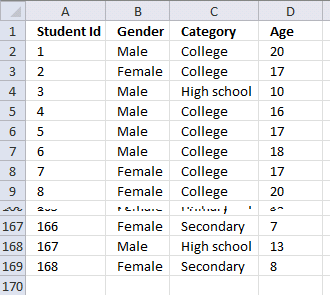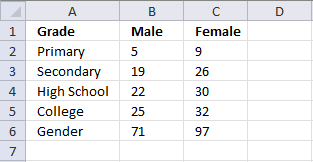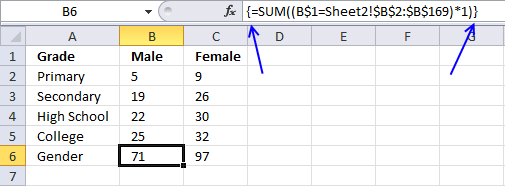Author: Oscar Cronquist Article last updated on July 30, 2017

Hi Oscar,
I have the following issues. Any suggestions.

Sheet 1 :
Primary
Secondary
High School
College
Gender

Sheet 2 :
Col A : Student Id
Col B: Gender : Here we specify whether the student is male or female
Col C : Here we specify category to which the student falls : Primary, secondary, high school, college
Col D : Age : To which age they are in

Problem :

In Sheet 1,
# I should give the count of students who are male and are in
* primary and they are between 4 to 6 years.
* Count of Secondary students and between 6 to 10 years ...

# I should give the count of students who are female and in
* primary and they are between 4 to 6 years.
* Count of Secondary students and between 6 to 10 years

Kindly let me know of any specific formula e that I can use.

Sheet 2 - Example dataSheet 1 - Count studentsFormula in cell B2:

=SUMPRODUCT((\$A2=Sheet2!\$C\$2:\$C\$169)*(B\$1=Sheet2!\$B\$2:\$B\$169))

You can copy this formula to cell range B2:C5. The formula takes advantage of both absolute and relative cell references . For this to work, copy the cell, not the formula in the cell or formula bar.

Example,

1. Select cell B2
2. Copy cell B2 (Ctrl + c)
3. Select cell range B2:C5
4. Paste (Ctrl + v)

Read more about absolute and relative cell references

Formula in cell B6:

=SUMPRODUCT((B\$1=Sheet2!\$B\$2:\$B\$169)*1)

If you are familiar with array formulas, use this array formula in cell B6:

=SUM((B\$1=Sheet2!\$B\$2:\$B\$169)*1)

The formula is smaller but needs to be entered as an array formula.

How to enter an array formula

1. Select cell B6
2. Paste formula to formula bar3. Press and hold CTRL + SHIFT
4. Press Enter simultaneously
5. Release all keys

If you did it right, the formula now begins and ends with curly brackets, like this:### Explaining formula in cell B2

=SUMPRODUCT((\$A2=Sheet2!\$C\$2:\$C\$169)*(B\$1=Sheet2!\$B\$2:\$B\$169))

Step 1 - Compare value in cell A2 (sheet1) to values in cell range C2:C169 (sheet2)

\$A2=Sheet2!\$C\$2:\$C\$169

becomes

"Primary"={"College"; "College"; "High school"; ... ; "Secondary"}

and returns

{FALSE; FALSE; FALSE; ... ; FALSE}

Step 2 - Compare value in cell B1 (sheet1) to values in cell range B2:B169 (sheet2)

B\$1=Sheet2!\$B\$2:\$B\$169

becomes

"Male"={"Male"; "Female"; "Male"; ... ; "Female"}

and returns

{"TRUE"; "FALSE"; "TRUE"; ... ; "FALSE"}

Step 3 - Multiplying arrays

(\$A2=Sheet2!\$C\$2:\$C\$169)*(B\$1=Sheet2!\$B\$2:\$B\$169)

becomes

("Primary"={"College"; "College"; "High school"; ... ; "Secondary"})*("Male"={"Male"; "Female"; "Male"; ... ; "Female"})

becomes

({FALSE; FALSE; FALSE; ... ; FALSE})*({"TRUE"; "FALSE"; "TRUE"; ... ; "FALSE"})

and returns

({0; 0; 0; ... ; 0})

Step 4 - Sum all values in the array

=SUMPRODUCT((\$A2=Sheet2!\$C\$2:\$C\$169)*(B\$1=Sheet2!\$B\$2:\$B\$169))

becomes

=SUMPRODUCT({0; 0; 0; ... ; 0})

and returns 5 in cell B2.

### Explaining formula in cell B6

=SUMPRODUCT((B\$1=Sheet2!\$B\$2:\$B\$169)*1)

Step 1 - Compare value in cell B1 (sheet1) to values in cell range B2:B169 (sheet2)

B\$1=Sheet2!\$B\$2:\$B\$169

becomes

"Male"={"Male"; "Female"; "Male"; .. ; "Female"}

and returns

{"TRUE"; "FALSE"; "TRUE"; .. ; "FALSE"}

Step 2 - Convert boolean values to numerical values

SUMPRODUCT function (and the sum function) can´t sum boolean values (TRUE, FALSE). Multiplying with 1 converts TRUE to 1 and FALSE to 0.

(B\$1=Sheet2!\$B\$2:\$B\$169)*1

becomes

{"TRUE"; "FALSE"; "TRUE"; .. ; "FALSE"}*1

and returns

{1; 0; 1; .. ; 0}

Step 3 - Sum values

SUMPRODUCT((B\$1=Sheet2!\$B\$2:\$B\$169)*1)

becomes

SUMPRODUCT({1; 0; 1; .. ; 0})

and returns 71 in cell B6.

### More to note

You can also use the SUMIF and SUMIFS function to count values. See this post:
Sum values between two dates with criteria